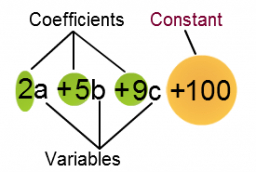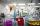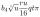# Equivalent expression

Pablo rewrites the expression 8y-24 as 8(y-3). Hana rewrites 8y-24 as 2(4y-12). Are Pablo’s and Hana’s expressions each equivalent to 8y-24? Explain your reasoning.

Result

x=

### Step-by-step explanation:Did you find an error or inaccuracy? Feel free to write us. Thank you!

Showing 1 comment:Dr Math
Yes, Pablo's and Hana's expression are both  equivalent to 8y-24  - see distributive property / law## Related math problems and questions:

• Distributive propertyVerify the distributive property a×(b+c)=(a×b)+(a*c) for the rational number a=5/8, b=7/4 and c=2/3
• Fractions and mixed numerals(a) Convert the following mixed numbers to improper fractions. i. 3 5/8 ii. 7 7/6 (b) Convert the following improper fraction to mixed number. i. 13/4 ii. 78/5 (c) Simplify these fractions to their lowest terms. i. 36/42 ii. 27/45 2. evaluate following ex
• Equivalent equationWhich equation, when solved, results in a different value of x than the other three? A. - (7)/(8) x - (3)/(4) = 20 Negative 7/8 x minus three-fourths = 20 B. (3)/(4) + (7)/(8) x= -20 Three-fourths + StartFraction 7 over 8 En
• Multiplication negativesJosh says that (4)(-7)(-1) is equal to (4)(7). Evangelina says (4)(-7)(-1) is equal to (-28)(-1) . Who is correct? Explain your reasoning.
• BoatsThree-quarters of boats are white, 1/7 are blue and 9 are red. How many boats do we have?
• Simple equation 324 = n • 27, solve for n
• Three friendsJohn, Peter, and Pablo each carried a 24 liters bucket full of water down the hill. After they reached the bottom, John's bucket was only 3/4 full, Peter's bucket was 2/3 full, and Pablo's was 1/6 full. How much liters of water did they spill altogether o
• Evaluate mixed expressionsWhich of the following is equal to 4 and 2 over 3 divided by 3 and 1 over 2? A. 4 and 2 over 3 times 3 and 2 over 1 B. 14 over 3 times 2 over 7 C. 14 over 3 times 7 over 2 D. 42 over 3 times 2 over 31
• TranslateTranslate the following mathematical statement into an algebraic expression or equation. 1. Nine less that the quotient of a number and 3 2. The ratio of 3 and the sum of 4 and an unknown number 3. Eighty is the product of 4 and g 4. The sum of x and 18 i
• SimplifySimplify expression - which expression is equivalent to: 3(m + 2) − 4(2m − 9)
• Crystal waterThe chemist wanted to check the content of water of crystallization of chromic potassium alum K2SO4 * Cr2 (SO4) 3 * 24 H2O, which was a long time in the laboratory. From 96.8 g of K2SO4 * Cr2 (SO4) 3 * 24 H2O prepared 979 cm3 solution of base.
• Expressions with variableThis is algebra. Let n represent an unknown number and write the following expressions: 1. 4 times the sum of 7 and the number x 2. 4 times 7 plus the number x 3. 7 less than the product of 4 and the number x 4. 7 times the quantity 4 more than the number
• ExpressionsExpression (3a-7).(3a+7) is identical with: ?
• Equivalent fractionsAre these two fractions equivalent -4/9 and 11/15?
• SelectSelect the statement that describes this expression: 5 x (3 + 4) - 4. a) 5 times the sum of 3 and 4, then subtract 4 b) 5 times 3 plus 4 minus 4 c) 5 more than the sum of 3 and 4, and then subtract 4 d) 5 times 3 plus the difference of 3 and 4 What is the
• Equivalent fractions 2Write the equivalent multiplication expression. 2 1/6÷3/4
• Evaluate 9Evaluate expression: 3 { 4 − 4 ( 5 − 12 ) } + 20